# What are variables in algebra?#### Everything You Need in One Place

Homework problems? Exam preparation? Trying to grasp a concept or just brushing up the basics? Our extensive help & practice library have got you covered.#### Learn and Practice With Ease

Our proven video lessons ease you through problems quickly, and you get tonnes of friendly practice on questions that trip students up on tests and finals.#### Instant and Unlimited Help

Our personalized learning platform enables you to instantly find the exact walkthrough to your specific type of question. Activate unlimited help now!

0/5
##### Intros
###### Lessons
1. Introduction to Variables in Algebra:
2. What are equations?
3. What are unknowns and how do you find out their value?
4. What are variables?
5. What are the equation models for the four operations?
6. How to write algebraic equations from sentences
0/19
##### Examples
###### Lessons
1. Unknowns in addition or subtraction equations
Draw the missing circles in the unknown box. Write the missing number in the equation.
1.2.3.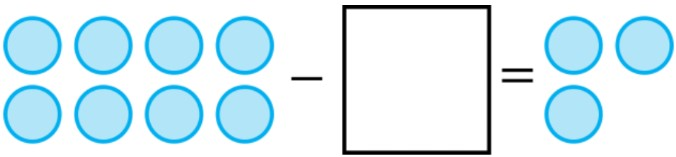4.2. Unknowns in multiplication or division equations
Finish drawing the circles to solve the equation. Trace around the circles to make equal groups.
1.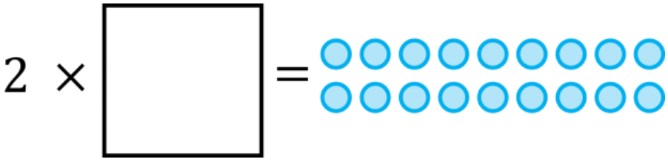2.3.4.3. Writing equations with variables for addition or subtraction
Write the equation with variables for the phrase:
1. 10 more than $h$ equals 64
2. 73 increased by $x$ equals 109
3. $b$ less than 8 equals 2
4. 24 decreased by $s$ equals 17
4. Writing equations with variables for multiplication or division
Write the equation with variables for the phrase:
1. the product of 4 and 6 equals $a$
2. 9 times $j$ equals 72
3. the quotient of 24 and 3 equals $c$
4. $k$ divided by 10 equals 5
5. Equations with variables: word problems
Write the equation for the word problem. Use the variable $x$ for the unknown value.
1. Allie picked 9 strawberries and Troye picked 8 strawberries. How many strawberries did they pick in total?
2. Brian got 24 on the quiz. Ellie got 2 points less than Brian. How many points did Ellie get on her quiz?
3. For which case should you use multiplication or division?
1. There are 5 red apples. There are 2 times as many green apples as red apples. How many green apples are there?

2. There are 8 red apples. There are 2 times as many red apples as green apples. How many green apples are there?
0%
##### Practice
###### Topic Notes

In this lesson, we will learn:

• The parts of a math equation for all four operations: addition, subtraction, multiplication, division
• How to do basic algebra: solving for unknowns (variables) in equations
• How to write algebra equations from word sentences by using equation models to help

Notes:

• A math equation can be thought of as a mathematical sentence which needs terms, an operation and an answer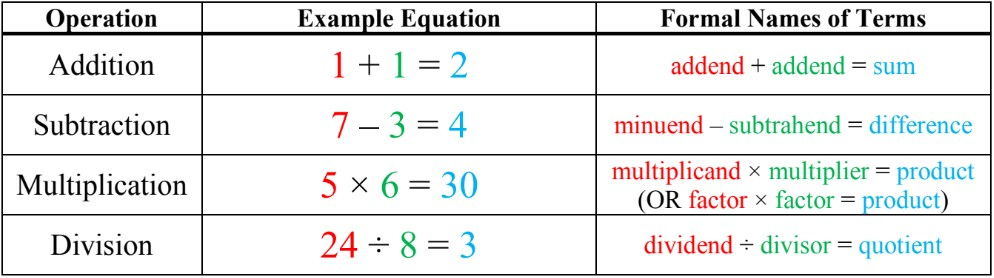• An unknown is a value that we don't know in an equation yet; we need to solve the equation to find the unknown through guess-and-check (knowing that values must be equal on both sides of the equal sign)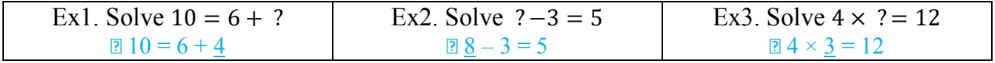• Algebra is a type of math where we will see BOTH numbers and letters (or symbols)!
• We use variables (letters or symbols) to represent unknown values: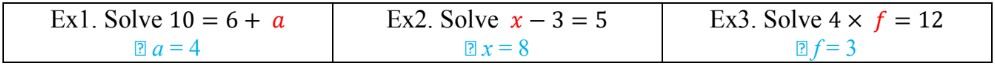• Equation models help identify all the terms when you're dealing with word sentences so that you can write an algebra equation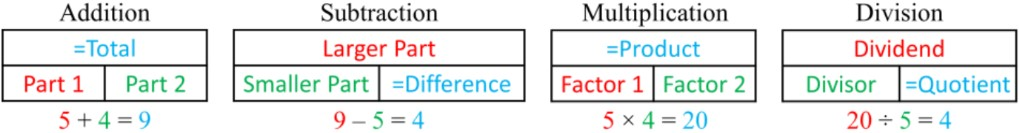• i.e. "36 added to 41 equals $y$." $\,$$\,$36 + 41 = $y$
• i.e. "15 less than $f$ equals 13." $\,$$\,$$f$ - 15 = 13
• i.e. "2 times as many as 7 is $m$." $\,$$\,$2 × 7 = $m$
• i.e. "$x$ is 3 times less than 15" $\,$$\,$$x$ = 15 ÷ 13

• Keywords (key phrases) for each operation include: